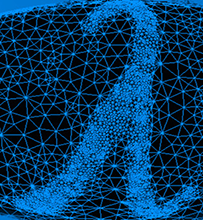Graph SparsificationThis series of talks was part of the Algorithmic Spectral Graph Theory Boot Camp. Videos for each talk are available through the links above.

Approximating a given graph by a graph with fewer edges or vertices is called sparsification. The notion of approximation that is most relevant to this workshop is the spectral one, in which two graphs are considered close if their Laplacian matrices are close as linear operators. It turns out that spectral approximations exist for every weighted undirected graph and can often be computed very quickly; in the past few years this has become a useful and versatile primitive in designing efficient graph algorithms.

We will survey constructions of (weighted) spectral sparsifiers in various parameter regimes (polylogarithmic degree, constant degree, and beyond) and mention their applications. These constructions involve viewing a graph in three different ways: combinatorially, as an electrical circuit, and as a bunch of vectors. We will then describe a more recent method which shows that unweighted sparsifiers exist many cases; it is based on viewing the subgraphs of a graph as polynomials, and exploiting real-rootedness properties of their averages. This last technique is rather general and can be used to solve other problems outside graph theory, such as the Kadison-Singer problem.

Time permitting, we will also discuss more practical, streaming algorithms for sparsification, as well as the important special case when the input graph is the complete graph (whose sparsifiers are the ubiquitous expander graphs).